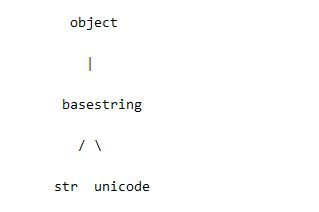# 《Python之禅》中对于Python编程过程中的一些建议

更新时间：2015年04月03日 11:01:59   作者：Satyajit Ranjeev我要评论

```#-----------------------------------------------------------------------
halve_evens_only = lambda nums: map(lambda i: i/2,\
filter(lambda i: not i%2, nums))
#-----------------------------------------------------------------------
def halve_evens_only(nums):
return [i/2 for i in nums if not i % 2]
```

```a, b = b, a
```

```a = [1,2,3,4,5]
>>> a[::2] # 以步长为2的增量迭代整个list对象
[1,3,5]
```

一个特殊的例子 `x[::-1]`用来反转x的实用语法。

```>>> a[::-1]
[5,4,3,2,1]
```

```def function(x, l=[]):     # 不要这么干
def function(x, l=None):    # 更好的一种方式
if l is None:
l = []
```

iteriterms 使用的是 generators，所以当迭代很大的序列是此方法更好

```d = {1: "1", 2: "2", 3: "3"}

for key, val in d.items()    # 调用items()后会构建一个完整的list对象

for key, val in d.iteritems()  # 只有在迭代时每请求一次才生成一个值
```

```if type(s) == type(""): ...
if type(seq) == list or \
type(seq) == tuple: ...
```

```if isinstance(s, basestring): ...
if isinstance(seq, (list, tuple)): ...
```

```>>> a=u'aaaa'
>>> print isinstance(a, basestring)
True
>>> print isinstance(a, str)
False
```python有各种各样的容器数据类型，在特定情况下选择python内建的容器如：list和dict。通常更多像如下方式使用：

```freqs = {}
for c in "abracadabra":
try:
freqs[c] += 1
except:
freqs[c] = 1

```

```freqs = {}
for c in "abracadabra":
freqs[c] = freqs.get(c, 0) + 1
```

```from collections import defaultdict
freqs = defaultdict(int)
for c in "abracadabra":
freqs[c] += 1
```

```namedtuple()    # 用指定的域创建元组子类的工厂函数
deque      # 类似list的容器，快速追加以及删除在序列的两端
Counter     # 统计哈希表的dict子类
OrderedDict      # 记录实体添加顺序的dict子类
defaultdict      # 调用工厂方法为key提供缺省值的dict子类
```

```__eq__(self, other)   # 定义相等操作的行为, ==.
__ne__(self, other)   # 定义不相等操作的行为, !=.
__lt__(self, other)   #定义小于操作的行为, <.
__gt__(self, other)   #定义不大于操作的行为, >.
__le__(self, other)   #定义小于等于操作的行为, <=.
__ge__(self, other)   #定义大于等于操作的行为, >=.

```

```x = 3 if (y == 1) else 2
```

```x = 3 if (y == 1) else 2 if (y == -1) else 1
```

```(func1 if y == 1 else func2)(arg1, arg2)
```

func1将被调用如果y等于1的话，反之func2被调用。两种情况下，arg1和arg2两个参数都将附带在相应的函数中。

```x = (class1 if y == 1 else class2)(arg1, arg2)
```

class1和class2是两个类

```class MyClass(object):
def __init__(self, a, b, c, d):
self.a, self.b, self.c, self.d = a, b, c, d

def __getitem__(self, item):
return getattr(self, item)

x = MyClass(10, 12, 22, 14)
```

```def __getitem__(self, item):
if item is Ellipsis:
return [self.a, self.b, self.c, self.d]
else:
return getattr(self, item)
```

```>>> x = MyClass(11, 34, 23, 12)
>>> x[...]
[11, 34, 23, 12]```